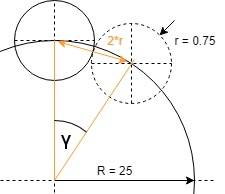ntb:motors_init

Initialization - Zero reference

The initialization for the axis motors is the same as Initialization - Zero reference with the additional motor for the TCP.

Mechanical Limits of the Tool Center Point Motor

The TCP rotation is limited by two metall pins. The maximum turn angle is calculated as follows:sin(γ/2) = r/R